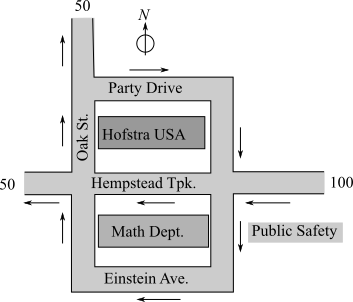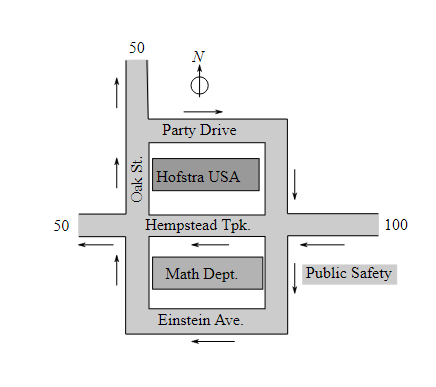Chapter 4.3, Problem 34EFinite Mathematics and Applied Cal...

7th Edition
Stefan Waner + 1 other
ISBN: 9781337274203

Solutions

Chapter
SectionFinite Mathematics and Applied Cal...

7th Edition
Stefan Waner + 1 other
ISBN: 9781337274203
Textbook Problem

Traffic Flow Officials of the town of Hempstead were planning to make Hempstead Turnpike and the surrounding streets into one-way streets to prepare for the opening of Hofstra USA. In an experiment, they restricted traffic flow along the streets near Hofstra as shown in the diagram. The numbers show traffic flow per minute and the arrows indicate the direction of traffic.a. Set up and solve the associated traffic flow problem with the following unknowns: x = Traffic per minute along the middle stretch of Oak St. y = Traffic per minute along the middle stretch of Hempstead Tpk. z = Traffic per minute along Einstein Ave. u = Traffic per minute along Party Drive.b. If 20 cars per minute drive along Party Drive, what is the traffic like along the middle stretch of Oak Street?c. If 20 vehicles per minute drive along Einstein Ave. and 90 vehicles per minute drive down the middle stretch of Hempstead Tpk., how many cars per minute drive along the middle stretch of Oak Street?d. If Einstein Avenue is deserted, what is the minimum traffic along the middle stretch of Hempstead Tpk.?

(a)

To determine

To calculate: The traffic flow across the given experiment done by restricting the flow along the streets as shown in the provided diagram.Explanation

Given Information:

The flow along the streets is as shown in diagram.

The unknowns are:

x is the traffic per minute along the middle stretch of oak St.

y is the traffic per minute along the middle stretch of Hempstead Tpk.

z is the traffic per minute along Einstein Ave.

u is the traffic per minute along Party drive.

Formula Used:

Elementary row operations

Type 1: Replace row Ri by aRi. Here, a is a non-zero number.

Type 2: Replace row Ri by aRi±bRj. Here, a is a non-zero number.

Gauss Jordan reduction method:

Step 1: Using type 1 operations, clear the fractions or decimals, if any.

Step 2: Select the first non-zero element of the first row as pivot.

Step 3: Using type 2 operations, use the pivot to clear its column.

Step 4: Select the first non-zero element in the second row as a pivot and clear its column.

Step 5: Turn all the selected pivot elements into a 1 using operations of type 1.

Calculation:

Consider the provided information,

x is the traffic per minute along the middle stretch of oak St.

y is the traffic per minute along the middle stretch of Hempstead Tpk.

z is the traffic per minute along Einstein Ave.

u is the traffic per minute along Party drive.

Equate the traffic in and traffic out at each intersection:

At intersection A

x=50+uxu=50

At intersection B

z+y=50+xx+y+z=50

At intersection C

100+u=y+zuyz=100yz+u=100

Consider the system of equation,

xu=50x+y+z=50yz+u=100

The augmented matrix for the given system of equations is,



Apply Gauss Jordan reduction method to get the solution of the given system of equation

(b)

To determine

To calculate: the traffic like along the middle stretch of ok street if 20 cars per minute drive along party drive when the flow across the given experiment done by restricting the flow along the streets as shown in the provided diagram.(c)

To determine

To calculate: the traffic like along the middle stretch of oak street if 20 cars per minute drive along Einstein ave and 90 cars per minute drive down the middle stretch of Hempstead Tpk.

The flow across the given experiment done by restricting the flow along the streets as shown in the provided diagram.(d)

To determine

To calculate: The minimum traffic along the middle stretch of Hempstead Tpk, if Einstein ave is deserted.

The flow across the given experiment done by restricting the flow along the streets as shown in the provided diagram.Still sussing out bartleby?

Check out a sample textbook solution.

See a sample solution

The Solution to Your Study Problems

Bartleby provides explanations to thousands of textbook problems written by our experts, many with advanced degrees!

Get Started

In Exercises 17-20, determine whether the point lies on the graph of the function. 17. (2, 3); g(x)=x21

Applied Calculus for the Managerial, Life, and Social Sciences: A Brief Approach

Prove that if then.

Elements Of Modern Algebra

Sometimes, Always, or Never: If {an} is increasing and bounded above, then {an} converges.

Study Guide for Stewart's Single Variable Calculus: Early Transcendentals, 8th

The curvature of at t = 0 is:

Study Guide for Stewart's Multivariable Calculus, 8th